Home | | Dynamics of Machines | Inertia Forces in a Reciprocating Engine, Considering the Weight of connecting Rod

# Inertia Forces in a Reciprocating Engine, Considering the Weight of connecting Rod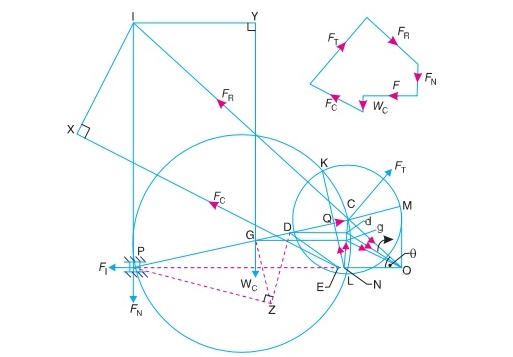In a reciprocating engine, let OC be the crank and PC, the connecting rod whose centre of gravity lies at G. The inertia forces in a reciprocating engine may be obtained graphically as discussed below:

INERTIA FORCES IN A RECIPROCATING ENGINE, CONSIDERING THE WEIGHT OF CONNECTING ROD

In a reciprocating engine, let OC be the crank and PC, the connecting rod whose centre of gravity lies at G. The inertia forces in a reciprocating engine may be obtained graphically as discussed below:

1. First of all, draw the acceleration diagram OCQN by KlienŌĆÖs construction. We know that the acceleration of the piston P with respect to O,acting in the direction from N to O. Therefore, the inertia force FI of the reciprocating parts will act in the opposite direction as shown in Fig. 15.22.Fig. 15.22. Inertia forces is reciprocating engine, considering the weight of connecting rod.

2. Replace the connecting rod by dynamically equivalent system of two masses as discussed in Art. 15.12. Let one of the masses be arbitrarily placed at P. To obtain the position of the other mass, draw GZ perpendicular to CP such that GZ = k, the radius of gyration of the connecting rod. Join PZ and from Z draw perpendicular to DZ which intersects CP at D. Now, D is the position of the second mass.

Note: The position of the second mass may also be obtained from the equation,

GP ├Ś GD   = k2

3. Locate the points G and D on NC which is the acceleration image of the connecting rod. This is done by drawing parallel lines from G and D to the line of stroke PO. Let these parallel lines intersect NC at g and d respectively. Join gO and dO. Therefore, acceleration of G with respect to O, in the direction from g to O,

aGO         = aG = Žē2 ├Ś gO

and acceleration of D with respect to O, in the direction from d to O,

From D, draw DE parallel to dO which intersects the line of stroke PO at E. Since the accelerating forces on the masses at P and D intersect at E, therefore their resultant must also pass through E. But their resultant is equal to the accelerang force on the rod, so that the line of action of the accelerating force on the rod, is given by a line drawn through E and parallel to gO, in the direc- tion from g to O. The inertia force of the connecting rod FC therefore acts through E and in the opposite direction as shown in Fig. 15.22. The inertia force of the connecting rod is given by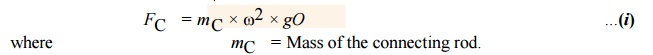A little consideration will show that the forces acting on the connecting rod are :

(a) Inertia force of the reciprocating parts (FI ) acting along the line of stroke PO,

(b) The side thrust between the crosshead and the guide bars (FN) acting at P and

right angles to line of stroke PO,

(c)  The weight of the connecting rod

(W C = mC.g), (d) Inertia force of the connecting rod (FC),

(e) The radial force (FR) acting through O and parallel to the crank OC, (f) The force (FT) acting perpen- dicular to the crank OC.

Now, produce the lines of action of FR and FN to intersect at a point I, known as instantaneous centre. From I draw I X and I Y , perpendicular to the lines of action of FC and W C. Taking moments about I, we have

FT ├Ś IC = FI ├Ś IP + FC ├Ś I X + W C ├Ś I Y ...(ii)

The value of FT may be obtained from this equation and from the force polygon as

shown in Fig. 15.22, the forces FN and FR may be calculated. We know that, torque exerted on the crankshaft to overcome the inertia of the moving parts = FT ├Ś OC

1 Analytical Method for Inertia Torque

The effect of the inertia of the connecting rod on the crankshaft torque may be obtained as discussed in the following steps: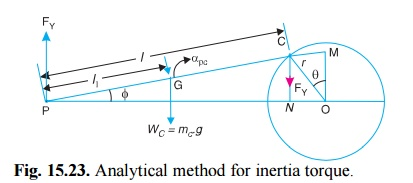Fig. 15.23. Analytical method for inertia torque.

1.The mass of the connecting rod (m C) is divided into two masses. One of the mass is placed at the crosshead pin P and the other at the crankpin C as shown in Fig. 15.23, so that the centre of gravity of these two masses coincides with the centre of gravity of the rod

G.

2. Since the inertia force due to the mass at C acts radially outwards along the crank OC, therefore the mass at C has no effect on the crankshaft torque.

3. The inertia force of the mass at P may be obtained as

follows: Let connecting rod,  mC  = Mass of the

l         = Length of the connecting rod,

l1        = Length of the centre of gravity of the connecting rod from P.4. In deriving the equation (ii) of the torque exerted on the crankshaft, it is assumed that one of the two masses is placed at C and the other at P. This assumption does not satisfy the condition for kinetically equivalent system of a rigid bar. Hence to compensate for it, a correcting torque is neces- sary whose value is given byThe correcting torque T' may be applied to the system by two equal and opposite forces FYacting through P and C. Therefore,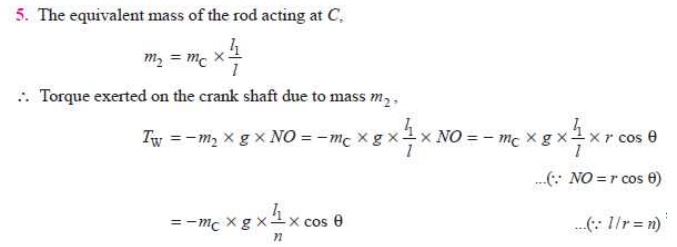Q.The crank and connecting rod lengths of an engine are 125 mm and 500 mm respectively. The mass of the connecting rod is 60 kg and its centre of gravity is 275 mm from the crosshead pin centre, the radius of gyration about centre of gravity being 150 mm.

If the engine speed is 600 r.p.m. for a crank position of 45┬░ from the inner dead centre, determine, using KlienŌĆÖs or any other construction 1. the acceleration of the piston; 2. the magni- tude, position and direction of inertia force due to the mass of the connecting rod.

Solution. Given : r = OC = 125 mm ; l = PC = 500 mm; m C = 60 kg ; PG = 275

mm ;

m C = 60 kg ; PG = 275 mm ; kG = 150 mm ; N = 600 r.p.m. or ’üĘ = 2’ü░ ├Ś 600/60 = 62.84 rad/s ; ’ü▒ = 45┬░

1. Acceleration of the piston

Let                   aP = Acceleration of the piston.

First of all, draw the configuration diagram OCP, as shown in Fig. 15.24, to some suitable scale, such that

OC = r = 125 mm ; PC = l = 500 mm ; and ’ü▒ = 45┬░.

Now, draw the KlienŌĆÖs acceleration diagram OCQN, as shown in Fig. 15.24, in the same manner as already discussed. By measurement,

NO = 90 mm = 0.09 m

’ü£ Acceleration of the piston,

aP = Žē2 ├Ś NO = (62.84)2 ├Ś 0.09 = 355.4 m/s Ans.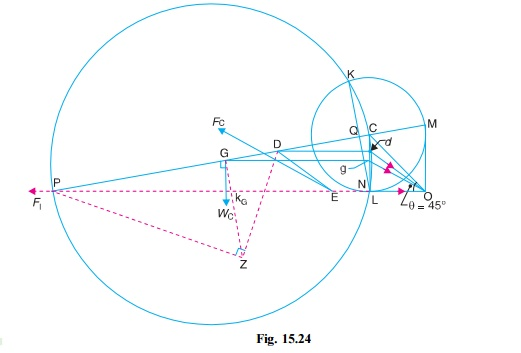2. The magnitude, position and direction of inertia force due to the mass of the connecting rod

The magnitude, postition and direction of the inertia force may be obtained as follows:

(i) Replace the connecting rod by dynamical equivalent system of two masses, assuming that one of the masses is placed at P and the other mass at D. The position of the point D is obtained as discussed in Art. 15.12.

(ii) Locate the points G and D on NC which is the acceleration image of the connecting rod. Let these points are g and d on NC. Join gO and dO. By measurement,

gO = 103 mm = 0.103 m

’ü£ Acceleration of G,   aG = Žē2 ├Ś gO, acting in the direction from g to O.

(iii) From point D, draw DE parallel to dO. Now E is the point through which the inertia force of the connecting rod passes. The magnitude of the inertia force of the connecting rod is given by

FC = mC ├Ś Žē2 ├Ś gO = 60 ├Ś (62.84)2 ├Ś 0.103 = 24 400 N = 24.4 kN Ans. (iv) From point E, draw a line parallel to gO, which shows the position of the

inertia force of

the connecting rod and acts in the opposite direction of gO.

Q. The following data refer to a steam engine:

Diameter of piston = 240 mm; stroke = 600 mm ; length of connecting rod = 1.5 m ; mass of reciprocating parts = 300 kg; mass of connecting rod = 250 kg; speed = 125 r.p.m ; centre of gravity of connecting rod from crank pin = 500 mm ; radius of gyration of the connecting rod about an axis through the centre of gravity = 650 mm.

Determine the magnitude and direction of the torque exerted on the crankshaft when the crank has turned through 30┬░ from inner dead centre.

Solution. Given : D = 240 mm = 0.24 m ; L = 600 mm or r = L/2 = 300 mm = 0.3 m ; l = 1.5 m ; mR = 300 kg ; mC = 250 kg ; N = 125 r.p.m. or Žē = 2ŽĆ ├Ś 25/60 = 13.1 rad/s ; GC = 500 mm = 0.5 m ;  kG = 650 mm = 0.65 m ; ╬Ė = 30┬░

The inertia torque on the crankshaft may be determined by graphical method or analytical method as discussed below:

1. Graphical method

First of all, draw the configuration diagram OCP, as shown in Fig. 15.25, to some suitable scale, such that

OC = r = 300 mm ; PC = l = 1.5 m ; and angle POC = ╬Ė = 30┬░.Now draw the KlienŌĆÖs acceleration diagram OCQN, as shown in Fig. 15.25, and complete the figure in the similar manner as discussed in Art. 15.14.

By measurement; NO = 0.28 m ; gO = 0.28 m ; IP = 1.03 m ; I X = 0.38 m ; I Y = 0.98 m, and IC = 1.7 m.

We know that inertia force of reciprocating parts,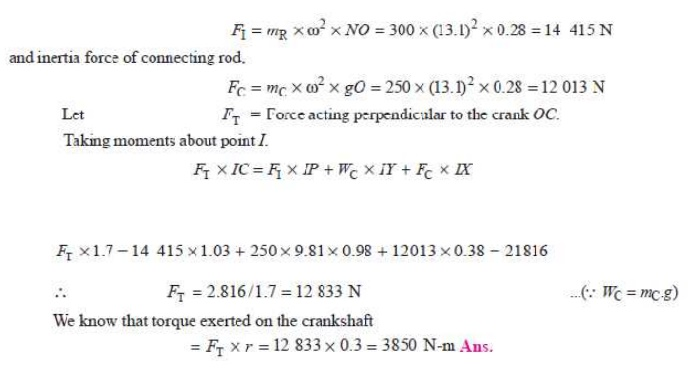Q. The connecting rod of an internal combustion engine is 225 mm long and has a mass 1.6 kg. The mass of the piston and gudgeon pin is 2.4 kg and the stroke is 150 mm. The cylinder bore is 112.5 mm. The centre of gravity of the connection rod is 150 mm from the small end. Its radius of gyration about the centre of gravity for oscillations in the plane of swing of the connect- ing rod is 87.5 mm. Determine the magnitude and direction of the resultant force on the crank pin when the crank is at 40┬░ and the piston is moving away from inner dead centre under an effective gas presure of 1.8 MN/m2. The engine speed is 1200 r.p.m.

Solution. Given : l = PC = 225 mm = 0.225 m; mC = 1.6 kg; m R = 2.4 kg; L = 150 mm or r = L/2 = 75 mm = 0.075 m ; D = 112.5 mm = 0.1125 m ; PG = 150 mm ; kG = 87.5 mm = 0.0875 m ; ╬Ė = 40┬░ ; p = 1.8 MN/m2 = 1.8 ├Ś 10 6 N/m2 ; N = 1200 r.p.m. or Žē = 2ŽĆ ├Ś 1200/60 = 125.7 rad/s

First of all, draw the configuration diagram OCP, as shown in Fig. 15.27 to some suitable scale, such that OC = r = 75 mm ; PC = l = 225 mm ; and ╬Ė = 40┬░.Now, draw the KlienŌĆÖs acceleration diagram OCQN. Complete the diagram in the same manner as discussed earlier. By measurement,

NO = 0.0625 m ; gO = 0.0685 m ; IC = 0.29 m ; IP = 0.24 m ; I Y = 0.148 m ; and IX = 0.08 m

We know that force due to gas pressure,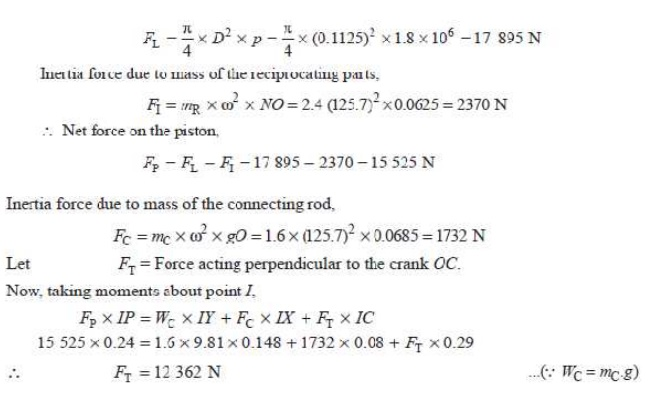Let us now find the values of FN and FR in magnitude and direction. Draw the force polygon as shown in Fig. 15.25.

By measurement, FN = 3550 N; and FR = 7550 N

The magnitude and direction of the resultant force on the crank pin is given by FQ , which is the resultant of FR and FT.

By measurement, FQ = 13 750 N  Ans.

Study Material, Lecturing Notes, Assignment, Reference, Wiki description explanation, brief detail
Mechanical : Dynamics of Machines : Force Analysis : Inertia Forces in a Reciprocating Engine, Considering the Weight of connecting Rod |